[BACK]Computer Modeling in Engineering & SciencesDOI: 10.32604/cmes.2021.015310

ARTICLE

A Pseudo-Spectral Scheme for Systems of Two-Point Boundary Value Problems with Left and Right Sided Fractional Derivatives and Related Integral Equations

1Department of Mathematics, Faculty of Science, Al-Azhar University, Cairo, Egypt
2Department of Mathematics, Faculty of Science, Cairo University, Giza, 12613, Egypt
3Department of Applied Mathematics, Physics Division, National Research Centre, Dokki, Cairo, 12622, Egypt
4Department of Computational Mathematics and Computer Science, Institute of Natural Sciences and Mathematics, Ural Federal University, Yekaterinburg, 620002, Russia
5Department of Mathematics, Faculty of Science, Benha University, Benha, 13511, Egypt
*Corresponding Author: M. A. Zaky. Email: ma.zaky@yahoo.com; zaky.nrc@gmail.com
Received: 08 December 2020; Accepted: 04 March 2021

Abstract: We target here to solve numerically a class of nonlinear fractional two-point boundary value problems involving left- and right-sided fractional derivatives. The main ingredient of the proposed method is to recast the problem into an equivalent system of weakly singular integral equations. Then, a Legendre-based spectral collocation method is developed for solving the transformed system. Therefore, we can make good use of the advantages of the Gauss quadrature rule. We present the construction and analysis of the collocation method. These results can be indirectly applied to solve fractional optimal control problems by considering the corresponding Euler–Lagrange equations. Two numerical examples are given to confirm the convergence analysis and robustness of the scheme.

Keywords: Spectral collocation method; weakly singular integral equations; two-point boundary value problems; convergence analysis

1  Introduction

Fractional-order differential operators have recently risen to prominence in the modelling of several processes. The mathematical models involving these operators have also attracted much attention, a survey of recent activity is given in . The issue we address in this paper is to construct and analyse a spectral collocation method to solve the following nonlinear system of Caputo fractional two-point boundary value problems:

{1CDzμu(z)=fL(z,u(z),v(z)),zCD1μv(z)=fR(z,u(z),v(z)),u(1)=u0,u(1)=u1,v(1)=v0,v(1)=v1,μ(1,2), (1)

where fR and fL:[1,1]×RR are continuous functions and satisfy the Lipschitz condition (64), and 1CDzμ and zCD1μ are the left- and right-sided Caputo fractional derivatives, respectively (see definition 2). In case of μ=2, then 1CDzμ and zCD1μ coincide with the usual second order derivative u″(z) and v″(z), and the system (1) recovers the integer-order system of two point boundary value problems.

Because the fractional-order differential operators are nonlocal with weakly singular kernels, the numerical discretization of the fractional models is more change than the classical schemes. There are several analytical schemes to solve fractional differential equations, such as the Green’s function method, the Mellin transform method, the Laplace transform method, the Fourier transform method, and so on . However, analytical methods are rare for most of fractional differential equations, e.g., with non linearities or linear equations with time-dependent coefficients. Hence, constructing efficient numerical approaches is of great importance in practical applications.

Many numerical schemes have been developed to solve the fractional differential equations, mostly with the finite element methods (e.g.,  and the references therein) and the finite difference methods (e.g.,  and the references therein). Since spectral methods are capable of providing high-order accurate numerical approximations with less degrees of freedoms , they have been widely used for numerical approximations of fractional differential equations  or its related integral equations . In particular, well designed spectral methods appear to be particularly attractive to tackle the difficulties associated with the weakly singular kernels of the fractional differential operators and the integral equations [38,39].

The system of fractional two-point boundary value problems (1) can be converted to an equivalent weakly singular nonlinear system of Volterra integral equations (15). The key idea of the presented approach is to solve (15) using the Legendre spectral collocation scheme. The aim of that convert to (15) is to approximate the related integral terms by the Gauss quadrature formula. The presented method has spectral convergence. This theoretical estimate is confirmed by two numerical test examples. Specifically, our strategies and contributions are highlighted as follows:

i)  The system of fractional two-point boundary value problems is recast into an equivalent weakly singular nonlinear system of Volterra integral equations.

ii)  The Legendre spectral collocation method is applied to the transformed equation.

iii)  The convergence analysis of the Legendre collocation method under the L2-norms is derived.

The structure of the paper is as follows. In Section 2, we introduce some necessary definitions, notations and lemmas. The Legendre spectral collocation scheme is presented in Section 3. The convergence analysis is provided in Section 4. In Section 5, numerical examples are performed to confirm the efficiency of the numerical method. A brief conclusion is highlighted in Section 6.

2  Mathematical Preliminaries

In this section, we provide some notations, definitions, and some useful lemmas about the fractional differential and integral operators  and the Jacobi polynomials.

Definition 1. Let t[1,1], for α>0, the left and right Riemann-Liouville fractional integrals of order μ are defined, respectively, as:

1Izμu(z)=1Γ(μ)1z(zτ)μ1u(τ)dτ,zI1μυ(z)=1Γ(μ)z1(τz)μ1υ(τ)dτ, (2)

where Γ(.) is the usual Gamma function.

Definition 2. The left- and right-sided Caputo fractional derivatives are defined as:

(1CDzμu)(z)=1Γ(mμ)1z(zτ)mμ1u(m)(τ)dτ,(zCD1μu)(z)=(1)mΓ(mμ)z1(τz)mμ1u(m)(τ)dτ, (3)

where , mN.

Definition 3. The left- and right-sided Riemann-Liouville fractional derivatives are defined as:

(1RLDzμu)(z)=1Γ(mμ)dmdzm1z(zτ)mμ1u(τ)dτ,(zRLD1μu)(z)=(1)mΓ(mμ)dmdzmz1(τz)mμ1u(τ)dτ. (4)

It is worthy to mention here that the left- and right-sided Caputo fractional derivatives satisfy the following fundamental properties

Theorem 1. There hold 

aIzα(aCDzμu(z))=aCDzμαu(z)j=μαμ1u(j)(a)Γ(j+αμ+1)(za)j+αμ, (5)

zITα(zCDTμu(z))=zCDTμαu(z)j=μαμ1u(j)(T)Γ(j+αμ+1)(Tz)j+αμ, (6)

where μα.

The following formulas introduce the relationship between the Riemann-Liouville and the Caputo fractional derivatives .

zDTμu(z)=zCDTμu(z)+j=0m1u(j)(T)Γ(jμ+1)(Tz)jμ. (8)

Let θ>0 and ϑ>1, then

aIzθ(za)ϑ=Γ(ϑ+1)Γ(ϑ+θ+1)(za)ϑ+θ,

and for θ(m1,m) with mN and ϑ0,

aCDzθ(za)ϑ={0,ϑm1, ϑN0,Γ(ϑ+1)Γ(ϑθ+1)(za)ϑθ,ϑ>m1, ϑR.

Now, we give some basic properties of the Jacobi polynomials and related Jacobi–Gauss interpolation. For ν, υ>1, the Jacobi polynomials Jiν,υ(ζ), ζΛ=[1,1] of degree i form a complete Lων,υ2(Λ) orthogonal system with the weight function ων,υ=(1ζ)ν(1+ζ)υ, i.e.,

ΛJiν,υ(ζ)Jjν,υ(ζ)ων,υ(ζ)dζ=γiν,υδi,j (9)

where, δi,j is the Kronecker function, and

γiν,υ=2ν+υ+1Γ(i+ν+1)Γ(i+υ+1)(2i+ν+υ+1)i!Γ(i+ν+υ+1). (10)

Denote PN(Λ) the space of all polynomials of degree less than or equal to N and {ω¯iν,υ,xiν,υ}i=0N are the set of weights and nodes of the Gauss-Jacobi interpolation. The associated Gauss-Jacobi integration formula can be written as:

ΛQ(x)ων,υ(x)dxi=0NQ(xi)ω¯iν,υ. (11)

The formula (11) is exact for any Q(x)P2N+1(Λ). Accordingly,

k=0NJiν,υ(xkν,υ)Jjν,υ(xkν,υ)ω¯kν,υ=γiν,υδi,j,0i+j2N+1. (12)

For any uC[1,1], the Gauss-Jacobi interpolating operator Jx,Nν,υ:C[1,1]PN(Λ) is determined uniquely by

Jx,Nν,υu(xjν,υ)=u(xjν,υ),0jN. (13)

The above condition indicates that Jx,Nν,υu=u, uPN. Consequently, since Jx,Nν,υuPN, then we can write

Jx,Nν,υu(x)=i=0Nυ^iν,υJiν,υ(x),where υ^iν,υ=1γiν,υj=0Nu(xj)Jjν,υ(xj)ω¯jν,υ. (14)

The Legendere polynomials can be obtained directly from the properties of the Jacobi polynomials by setting ν=υ=0 as Li(x)=Ji0,0(x). In the following sections, we drop the parameters ν and υ whenever ν=υ=0.

3  The Pseudo-Spectral Method

We consider the system of two-point fractional boundary value problems (1) with homogeneous boundary conditions. There is no loss of generality since this can always be accomplished by a simple change of variables.

{1CDzμu(z)=fL(z,u(z),v(z)),zCD1μv(z)=fR(z,u(z),v(z)),u(1)=u(1)=v(1)=v(1)=0,μ(1,2). (15)

The above system is equivalent to the following system of weakly singular integral equations:

{u(x)=1Γ(μ)1x(xσ)μ1fL(σ,u(σ),υ(σ))dσ,υ(x)=1Γ(μ)x1(σx)μ1fR(σ,u(σ),υ(σ))dσ. (16)

The variable transformations σ1(x,ζ)=x+12ζ1x2 and σ2(x,ζ)=x+12x12ζ are used in the first and second equations of the system (16) to convert the intervals (1,x) and (x,1) to the unit interval Λ as follows

u(x)=1Γ(μ)(1+x2)μ11(1ζ)μ1fL(σ1(x,ζ),u(σ1(x,ζ)),v(σ1(x,ζ))dζ, (17)

v(x)=1Γ(μ)(1x2)μ11(1+ζ)μ1fR(σ2(x,ζ),u(σ2(x,ζ)),v(σ2(x,ζ)))dζ. (18)

The Legendre spectral collocation scheme for (17) and (18) is to seek uN(x) and vN(x)PN(Λ) with N1, such that

uN(x)=1Γ(μ)Jx,N[(x+12)μ11(1ζ)μ1Jζ,Nμ1,0fL(σ1(x,ζ),uN(σ1(x,ζ)),vN(σ1(x,ζ))dζ] (19)

vN(x)=1Γ(μ)Jx,N[(1x2)μ11(1+ζ)μ1Jζ,N0,μ1fR(σ2(x,ζ),uN(σ2(x,ζ)),vN(σ2(x,ζ)))dζ]. (20)

We now describe the implementation procedure of (19), (20) in detail. We consider the following Legendre approximations

uN(x)=r=0Nu^rLr(x),Jx,NJζ,Nμ1,0((1+x2)μfL(σ1(x,ζ),uN(σ1(x,ζ)),vN(σ1(x,ζ)))=q=0Nq=0Nρ^q,qLq(x)Jqμ1,0(ζ), (21)

and

vN(x)=s=0Nv^sLs(x),Jx,NJs,N0,μ1((1x2)μfR(σ2(x,ζ),uN(σ2(x,ζ)),vN(σ2(x,ζ))))=r=0Nr=0Nξ^r,rLr(x)Jr0,μ1(ζ). (22)

Then, by (21) and (9) direct computations lead to

1Γ(μ)11(1ζ)μ1Jx,NJζ,Nμ1,0((1+x2)μfL(σ1(x,ζ),uN(σ1(x,ζ)),vN(σ1(x,ζ))))dζ=1Γ(μ)q=0Nq=0Nρ^q,qLq(x)11(1ζ)μ1Jqμ1,0(ζ)dζ=2μΓ(μ+1)q=0Nρ^q,0Lq(x). (23)

Applying (12) to (21), one can verify readily that

ρ^q,0=μ(2q+1)2μ+1i=0Nj=0N(xi+12)μ×fL(σ1(xi,ζjμ1,0),uN(σ1(xi,ζjμ1,0)),vN(σ1(xi,ζjμ1,0)))Lq(xi)ω¯iω¯jμ1,0. (24)

Similarly

1Γ(μ)11(1+s)μ1Jx,NJζ,N0,μ1((1x2)μfR(σ2(x,ζ),uN(σ2(x,ζ)),vN(σ2(x,ζ)))dζ=1Γ(μ)r=0Nr=0Nξ^r,rLr(x)11(1+ζ)μ1Jr0,μ1(s)dζ=2μΓ(μ+1)r=0Nξ^r,0Lr(x). (25)

Using (12)(22) yields

ξ^r,0=μ(2r+1)2μ+1i=0Nj=0N(1xi2)μ×fR(σ2(xi,ζj0,μ1),uN(σ2(xi,ζj0,μ1)),vN(σ2(xi,ζj0,μ1))Lr(xi)ω¯iω¯j0,μ1. (26)

Hence, by using (17)(26) we deduce that

i=0Nu^iLi(x)=2μΓ(μ+1)i=0Nρ^i,0Li(x), (27)

i=0Nv^iLi(x)=2μΓ(μ+1)i=0Nξ^i,0Li(x). (28)

Finally, using (9) we obtain

u^i=2μΓ(μ+1)ρ^i,0,0iN, (29)

v^i=2μΓ(μ+1)ξ^i,0,0iN. (30)

This system of equations can be solved for u^i and v^i. Then by using (21) and (22), we obtain an approximate solution for the problem (1).

4  Convergence Analysis

4.1 Auxiliary Lemmas

In this section, we introduce some functional spaces. We denote xmg(x) to be the mth derivative of g i.e., xmg(x):=dmgdxm(x). We also denote the Lων,μ2(Λ) inner product and norm by

(g,h)ων,μ:=Λg(x)h(x)ων,μdx, (31)

gων,μ:=(Λ|g(x)|2ων,μdx)12. (32)

Definition 4. Let s1 be an integer. The Sobolev space Hων,μs is defined as

Hων,μs(Λ):={gLων,μ2(Λ):xmgLων,μ2(Λ),0ms}, (33)

with the inner product and norm

(g,v)Hων,μs=m=0s(xmg,xmv)ων,μ, (34)

gHων,μs=(g,v)Hων,μs12. (35)

Definition 5. For a non-negative integer s. The weighted Jacobi non-uniformly Sobolev space Bων,μs is defined as

Bων,μs(Λ):={g:xmgLων+m,μ+m2(Λ),0ms}. (36)

with the inner product, norm, and semi-norm

(g,v)Bων,μs=m=0s(xmg,xmv)ων+m,μ+m, (37)

gBων,μs=(g,g)Bων+m,μ+ms12. (38)

In particular, L2(Λ)=Bω0,00(Λ) and .=.ω0,0. It is obvious that Hων,μs(Λ) is a subspace of Bων,μs(Λ), that is

uBων,μscuHων,μs.

The space L(Λ) is defined with the norm

u=esssupxΛ|u(x)|. (39)

Lemma 1. (cf. ) Let ν,μ>1, then for any uBων,μs(Λ) with s1 and 0ksN+1,

xk(uJx,Nν,μu)ων+k,μ+kcNksxsuων+k,μ+k, (40)

where Jx,Nν,μ is the Gauss-Jacobi interpolation operator.

Lemma 2. (cf. ) For any uHs(Λ) with 0sN+1,

uJx,NucN34sxsu. (41)

Lemma 3. (cf. ) Let {Fi(x)}i=0N be the Nth interpolation Lagrange polynomials related to the N + 1 Gauss points of the Jacobi polynomial. Then,

JNν,μ:=maxxΛi=0N|Fi(x)|={O(logN),1<ν,μ12,O(Nγ+12),γ=max(ν,μ),otherwise. (42)

Let ζiμ1,0 be the Gauss-Jacobi nodes in Λ and σ1,iμ1,0=σ1(x,ζiμ1,0). The mapped Gauss-Jacobi interpolating operator xJ~σ,Nμ1,0:C(1,x)PN(1,x) is given as

xJ~σ,Nμ1,0u(σiμ1,0)=u(σiμ1,0),0iN. (43)

Hence

xJ~σ,Nμ1,0u(σiμ1,0)=u(σiμ1,0)=u(σ1(x,ζiμ1,0))=xJσ,Nμ1,0u(σ1(x,ζiμ1,0)), (44)

and

xJ~σ,Nμ1,0u(σ)=Jζ,Nμ1,0u(σ1(x,ζ))|ζ=2σ11+x+1x1+x. (45)

It is not difficult to obtain the following results

1x(xσ)μ1xJ~σ,Nμ1,0fL(σ,u(σ),v(σ))dσ=(1+x2)μ11(1ζ)μ1Jζ,Nμ1,0fL(σ1(x,ζ),u(σ1(x,ζ)),v(σ1(x,ζ)))dζ=(1+x2)μj=0NfL(σ1(x,ζjμ1,0),u(σ1(x,ζjμ1,0)),v(σ1(x,ζjμ1,0)))ω¯jμ1,0=(1+x2)μj=0NfL(σ1,jμ1,0,u(σ1,jμ1,0),v(σ1,jμ1,0))ω¯jμ1,0. (46)

Similarly

1x(xσ)μ1(xJ~σ,Nμ1,0fL(σ,u(σ),v(σ)))2dσ=(1+x2)μj=0NfL2(σ1,jμ1,0,u(σ1,jμ1,0),v(σ1,jμ1,0))ω¯jμ1,0. (47)

We denote J to be the identity operator. Then, for any 1sN+1 we have

1x(xσ)μ1|JxJ~σ,Nμ1,0u(σ)|2dσ=(1+x2)μ11(1ζ)μ1|(JJζ,Nμ1,0)u(σ1(x,ζ))|2dζcN2s(1+x2)μ11(1ζ)μ+s1(1+ζ)s|ζsu(σ1(x,ζ))|2dζ=cN2s1x(xσ)μ+s1(1+σ)s|ζsu(σ)|2dσ (48)

Let ζi0,μ1 be the Jacobi-Gauss nodes in Λ and ζ2,i0,μ1=σ2(x,ζi0,μ1). The mapped Jacobi-Gauss interpolation operator xJ~ζ,N0,μ1:C(x,1)PN(x,1) is defined by

xJ~σ,N0,μ1v(ζi0,μ1)=v(ζi0,μ1),0iN. (49)

Hence,

xJ~σ,N0,μ1v(ζi0,μ1)=v(ζi0,μ1)=v(σ2(x,ζi0,μ1))=xJζ,N0,μ1v(σ2(x,ζi0,μ1)) (50)

and

xJ~ζ,N0,μ1v(ζ)=Jζ,N0,μ1v(σ2(x,ζ))|ζ=2σ21x1+x1x (51)

We can also derive the following results

x1(σx)μ1xJ~σ,N0,μ1fR(σ,u(σ),v(σ))dσ=(1x2)μ11(1+ζ)μ1Jζ,N0,μ1fR(σ2(x,ζ),u(σ2(x,ζ)),v(σ2(x,ζ)))dζ=(1x2)μj=0NfR(σ2(x,ζj0,μ1),u(σ2(x,ζj0,μ1),v(σ2(x,ζj0,μ1)))ω¯j0,μ1=(1x2)μj=0NfR(σ2,j0,μ1,u(σ2,j0,μ1),v(σ2,j0,μ1))ω¯j0,μ1. (52)

Similarly

x1(σx)μ1(xJ~σ,N0,μ1fR(σ,u(σ),v(σ)))2dζ=(1x2)μj=0NfR2(σ2,j0,μ1,u(σ2,j0,μ1),v(σ2,j0,μ1))ω¯j0,μ1, (53)

and

x1(σx)μ1|JxJ~σ,N0,μ1v(σ)|2dσ=(1x2)μ11(1+ζ)μ1|(JJζ,N0,μ1)v(σ2(x,ζ))|2dζcN2s(1x2)μ11(1+ζ)μ+s1(1ζ)s|ζsv(σ2(x,ζ))|2dζ=cN2sx1(σx)μ+s1(1σ)s|ζsv(σ)|2dσ. (54)

4.2 Error Analysis in L2-Norm

In this section, we analyze the numerical errors of the systems (19) and (20). Let E = |Eu|+|Ev|, where Eu = uuN, Ev = vvN and denote by J to be the identity operator.

Lemma 4. The following inequality holds

EEu+Evi=16Ei (55)

where

E1=u(x)Jx,Nu(x),E2=v(x)Jx,Nv(x),E3=1Γ(μ)Jx,N1x(xσ)μ1(JxJ¯σ,Nμ1,0)fL(σ,u(σ),v(σ))dσ,E4=1Γ(μ)Jx,Nx1(σx)μ1(JxJ¯σ,N0,μ1)fR(σ,u(σ),v(σ))dσ,E5=1Γ(μ)Jx,N1x(xσ)μ1xJ¯σ,Nμ1,0(fL(σ,u(σ),v(σ))fL(σ,uN(σ),vN(σ)))dσ,E6=1Γ(μ)Jx,Nx1(σx)μ1xJ¯σ,N0,μ1(fR(σ,u(σ),v(σ))fR(σ,uN(σ),vN(σ)))dσ.

Proof. It follows from (16) and (46) that

Jx,Nu(x)=1Γ(μ)Jx,N1x(xσ)μ1fL(σ,u(σ),v(σ))dσ, (56)

uN(x)=1Γ(μ)Jx,N1x(xσ)μ1xJ¯σ,Nμ1,0fL(σ,uN(σ),vN(σ))dσ. (57)

Subtracting (57) from (56) yields

Jx,Nu(x)uN(x)=1Γ(μ)Jx,N1x(xσ)μ1×(fL(σ,u(σ),v(σ))xJ¯σ,Nμ1,0fL(σ,uN(σ),vN(σ))dσ) (58)

Similarly, from (16) and (52) we deduce that

Jx,Nv(x)=1Γ(μ)Jx,Nx1(σx)μ1fR(σ,u(σ),v(σ))dσ, (59)

vN(x)=1Γ(μ)Jx,Nx1(σx)μ1xJ¯σ,N0,μ1fR(σ,uN(σ),vN(σ))dσ. (60)

Subtracting (60) from (59) yields

Jx,Nv(x)vN(x)=1Γ(μ)Jx,Nx1(σx)μ1×(fR(σ,u(σ),v(σ))xJ¯σ,N0,μ1fR(σ,uN(σ),vN(σ))dσ), (61)

and adding (58) and (61) yields

Jx,Nu(x)uN(x)+Jx,Nv(x)vN(x)=1Γ(μ)Jx,N1x(xσ)μ1(fL(σ,u(σ),v(σ))xJ¯σ,Nμ1,0fL(σ,uN(σ),vN(σ))dσ)+1Γ(μ)Jx,Nx1(σx)μ1(fR(σ,u(σ),v(σ))xJ¯σ,N0,μ1fR((σ,uN(σ),vN(σ))dσ). (62)

The above equation can be rewritten as

Jx,Nu(x)uN(x)+Jx,Nv(x)vN(x)=1Γ(μ)Jx,N1x(xσ)μ1(JxJ¯σ,Nμ1,0)fL(σ,u(σ),v(σ))dσ+1Γ(μ)Jx,N1x(xσ)μ1xJ¯σ,Nμ1,0(fL(σ,u(σ),v(σ))fL(σ,uN(σ),vN(σ)))dσ+1Γ(μ)Jx,Nx1(σx)μ1(JxJ¯σ,N0,μ1)fR(σ,u(σ),v(σ))dσ+1Γ(μ)Jx,Nx1(σx)μ1xJ¯σ,N0,μ1(fR(σ,u(σ),v(σ))fR(σ,uN(σ),vN(σ)))dσ. (63)

The desired result follows immediately from the above.

Throughout this section, we suppose that fL and fR fulfil the Lipschitz conditions

|fL(z,u1(z),v1(z))fL(z,u2(z),v2(z))|L11|u1u2|+L11|v1v2|,|fr(z,u1(z),v1(z))fr(z,u2(z),v2(z))|L22|u1u2|+L22|v1v2|, (64)

with the Lipschitz positive constants L11 and L22, are chosen such that

L11+L22Γ(λ+1)2λ. (65)

Theorem 2. Let uN(x) and vN(x) be the solutions of the systems of Eqs. (17) and (18), respectively, uBωs,ss(Λ) and vBωs,ss(Λ), with integer 1sN+1 and N1. Then we have the following estimate

Eu+EvcNs(xsuωs,s+xsvωs,s+xsfL(,u)),v())ωμ+s1,s+xsfR(,u)),v())ωs,μ+s1). (66)

Proof. Using Lemma (4), we get

E1cNsxsu(x), (67)

and

E2cNsxsv(x). (68)

We now estimate E3. Using the Gauss-Legendre integration formula (11), we have

E3=1Γ(μ)Jx,N1x(xσ)μ1(JxJ¯σ,Nμ1,0)fL(σ,u(σ),v(σ))dσ=[1Γ(μ)11(Jx,N1x(xσ)μ1(JxJ¯σ,Nμ1,0)fL(σ,u(σ),v(σ))dσ)2dx]12=[1Γ(μ)j=0Nωj¯(1xj(xjσ)μ1(JxjJ¯σ,Nμ1,0)fL(σ,u(σ),v(σ))dσ)2]12, (69)

using the Cauchy-Schwarz inequality, we further get

E3[1Γ(μ)j=0Nωj¯1xj(xjσ)μ1dσ1xj(xjσ)μ1|(JxjJ¯σ,Nμ1,0)fL(σ,u(σ),v(σ))|2dσ]12[1Γ(μ+1)j=0Nωj¯(xj+1)μ1xj(xjσ)μ1|(JxjJ¯σ,Nμ1,0)fL(σ,u(σ),v(σ))|2dσ]12, (70)

making use of (48) leads to

E3cNsmax0jN[1xj(xjσ)μ+s1(1+σ)s|σsfL(σ,u(σ),v(σ))|2dσ]12cNsσsfL(σ,u(σ),v(σ))ωμ+s1,s. (71)

E4=1Γ(μ)Jx,Nx1(σx)μ1(JxJ¯σ,N0,μ1)fR(σ,u(σ),v(σ))dσcNsσsfR(σ,u(σ),v(σ))ωs,μ+s12. (72)

We now estimate E5. Making use of the Gauss-Legendre integration (11) and (46), we have

E51Γ(μ)Jx,N1x(xσ)μ1xJ¯σ,Nμ1,0(fL(σ,u(σ),v(σ))fL(σ,uN(σ),vN(σ)))dσ=1Γ(μ)[11(Jx,N1x(xσ)μ1xJ¯σ,Nμ1,0(fL(σ,u(σ),v(σ))fL(σ,uN(σ),vN(σ)))dσ)2dx]121Γ(μ)[j=0Nωj¯(1xj(xjσ)μ1xjJ¯σ,Nμ1,0(fL(σ,u(σ),v(σ))fL(σ,uN(σ),vN(σ)))dσ)2]121Γ(μ)[j=0Nωj¯1xj(xjσ)μ1dσ1xj(xjσ)μ1|xjJ¯σ,Nμ1,0(fL(σ,u(σ),v(σ))fL(σ,uN(σ),vN(σ)))|2dσ]121Γ(μ)[j=0Nωj¯(xj+1)μμ1xj(xjσ)μ1|xjJ¯σ,Nμ1,0(fL(σ,u(σ),v(σ))fL(σ,uN(σ),vN(σ)))|2dσ]12.

Using (47), we obtain

E51Γ(μ)[j=0Nωj¯(xj+1)2μ2μμk=0N|(fL(σ1,kμ1,0,u(σ1,kμ1,0),v(σ1,kμ1,0))fL(σkμ1,0,uN(σ1,kμ1,0),vN(σ1,kμ1,0)))|2ω¯kμ1,0]12.

Using (46) and the Lipschitz condition, we get

E51Γ(μ)[j=0Nωj¯(xj+1)2μ2μμk=0Nω¯kμ1,0|L11|u(σ1,kμ1,0)uN(σ1,kμ1,0)|+L12|v(σ1,kμ1,0)vN(σ1,kμ1,0)||2]12,

For xj(1,1), one can show that

j=0Nωj(1xj)μ83,μ(1,2),

hence,

E5L11Γ(μ)[j=0Nωj¯(xj+1)μμ1xj(xjσ)μ1|xjJ¯σ,N0,μ1(|u(σ)uN(σ)|+|v(σ)vN(σ)|)|2dσ]128μ/3L11Γ(μ+1)max0jN[1xj(xjσ)μ1|xjJ¯σ,N0,μ1(|u(σ)uN(σ)|+|v(σ)vN(σ)|)|2dσ]128μ/3L11Γ(μ+1)max0jN[(1xj(xjσ)μ1(|xjJ¯σ,N0,μ1u(σ)u(σ)|+|xjJ¯σ,N0,μ1v(σ)v(σ)|)2dσ)12+(1xj(xjσ)μ1(|u(σ)uN(σ)|+|v(σ)vN(σ)|)2dσ)12]cNsxsuωs,s+cNsxsvωs,s+8μ/3L11Γ(μ+1)(Eu+Ev).

Similarly, we can deduce that

E6cNsxsuωs,s+cNsxsvωs,s+8μ/3L22Γ(μ+1)(Eu+Ev).

Using the condition (65) and knowing that 4μ3×2μ1, we obtain the desired result.

5  Numerical Results

In this section, two numerical examples are provided to illustrate the efficiency and applicability of the proposed method.

Example 1. We consider the following system of fractional differential equations:

{1CDzμu(z)=fL(z,u(z),v(z)),zCD1μv(z)=fR(z,u(z),v(z)),z(0,1)μ(1,2), (73)

The boundary conditions are chosen such that the exact solution is given by u(z) = (1+z)3.5 and v(z) = (1 − z)2.5. In Figs. 1 and 2 we list the L2-error of the approximate solutions uN and vN, respectively, in log scale against various N and μ. In Tab. 1, we list the maximum absolute errors for different values of N and μ.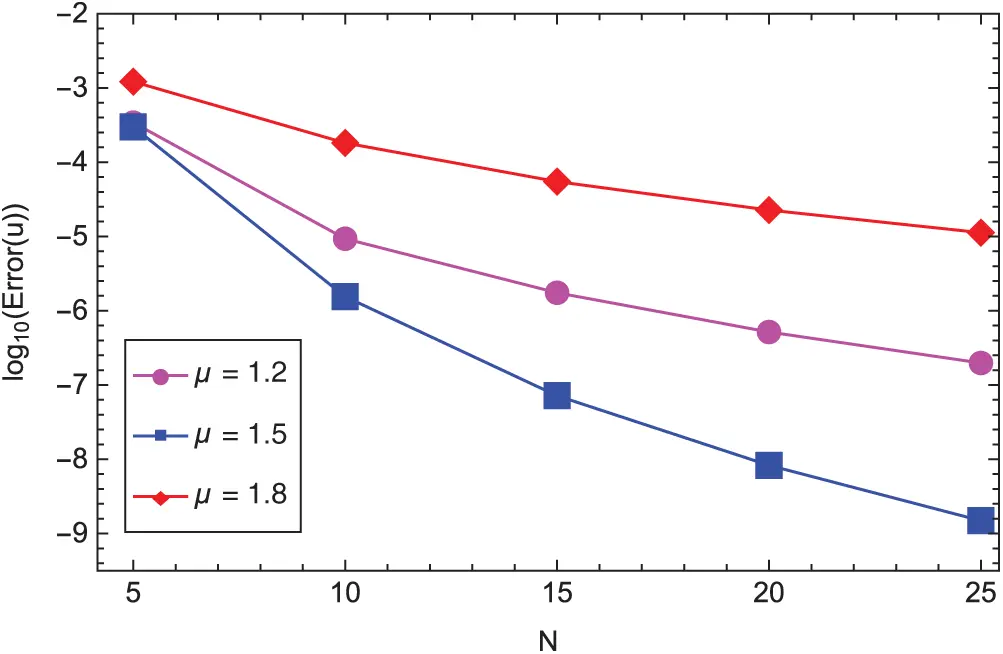Figure 1: The L2-error of the approximate solution uN in log scale vs. various N and μ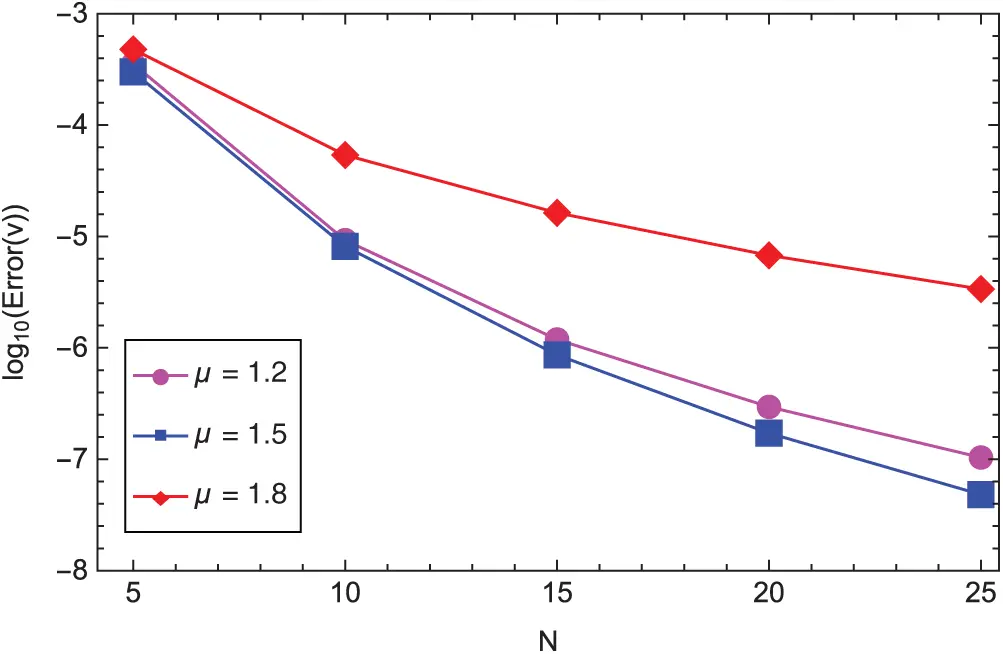Figure 2: The L2-error of the approximate solution vN in log scale vs. various N and μ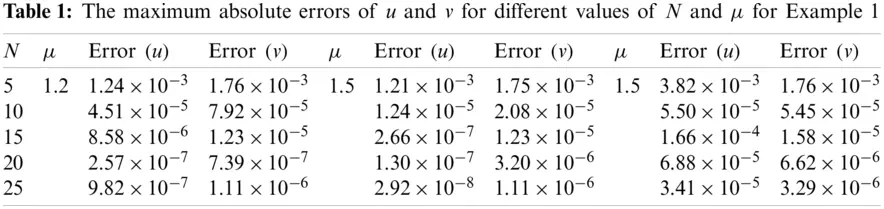Example 2. We consider the following system of fractional differential equations: :

{0CDtαx(t)=sinx(t)12λ(t)+sin(1+t2)2t2αΓ(3α),tCD1αλ(t)=2e1+t2+x(t)+2e2(1+t2+x(t))+λ(t)cosx(t),x(0)=1,x(0)=0,λ(1)=λ(1)=0, (74)

In Tab. 2, we compare our results with those reported in . These results indicate that the proposed spectral method is more accurate than the Ritz method . In Tab. 3, we list the maximum absolute errors for different values of N and α. We see in these tables that the results are accurate for even small choices of N. Moreover, to demonstrate the convergence of the proposed method, Figs. 3 and 4 present the logarithmic graphs of L-errors of the approximate solutions xN and λN, respectively, for various values of N and α. Clearly, the numerical errors decay as N increases.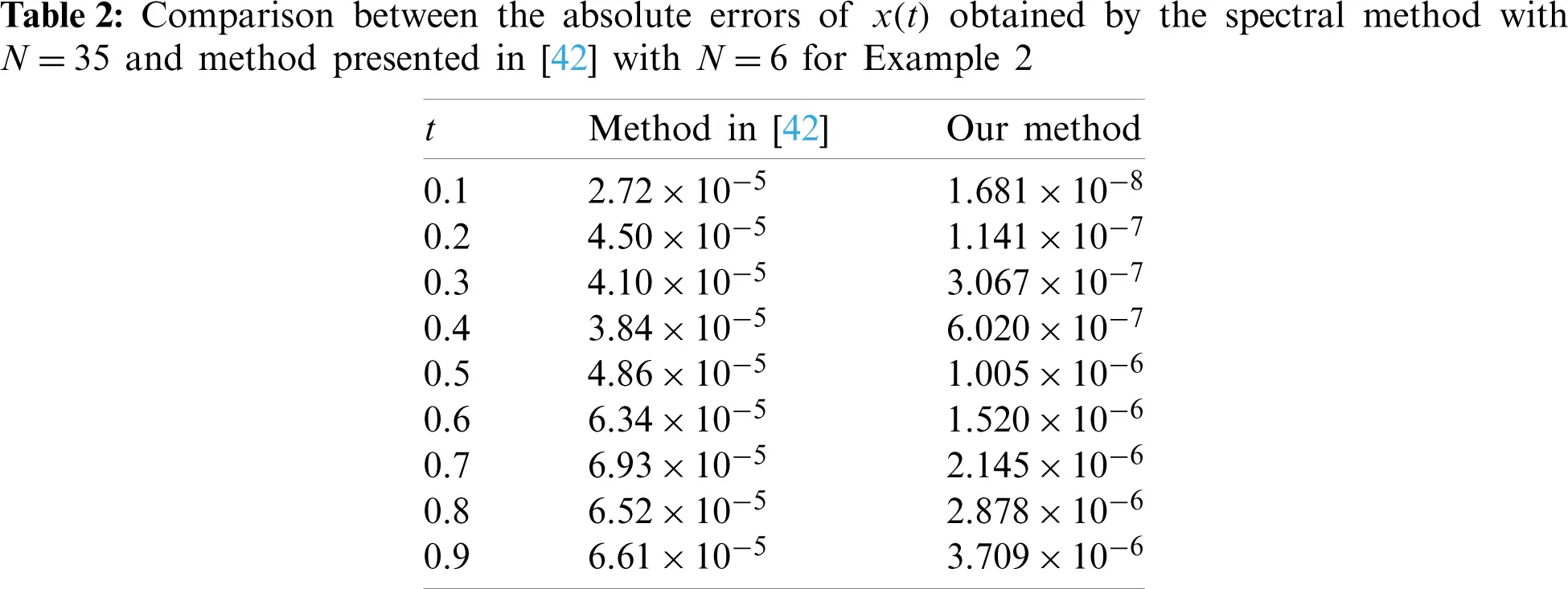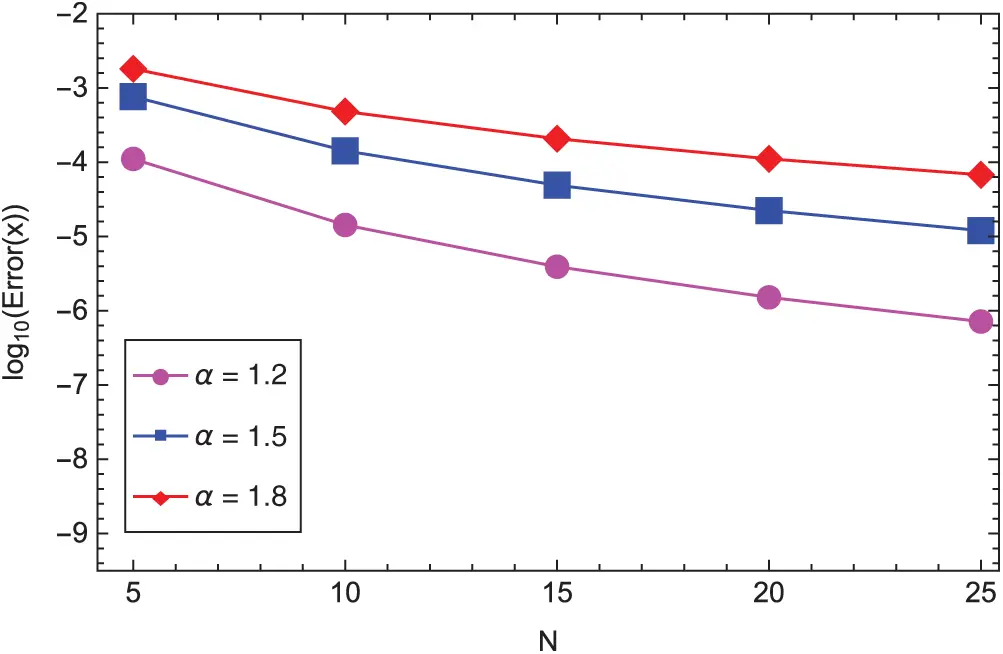Figure 3: The L-error of the approximate solution xN in log scale vs. various N and α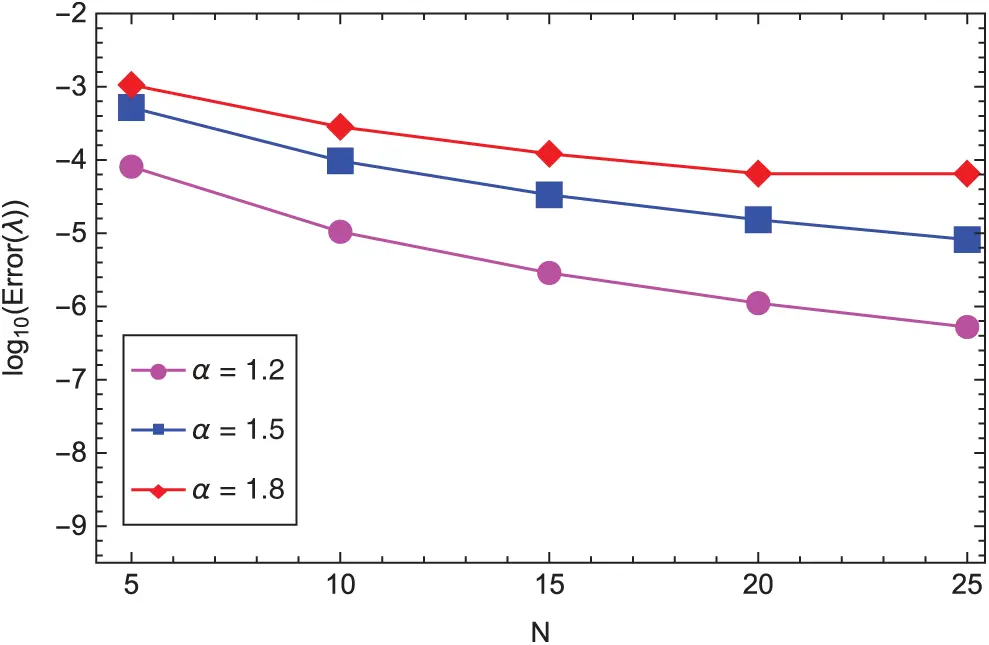Figure 4: The L-error of the approximate solution λN in log scale vs. various N and α

6  Conclusion

Numerically solving a class of nonlinear fractional two-point boundary value problems involving left- and right-sided fractional derivatives was fulfilled as a target of that work. The way to achieve that was done indirectly by recasting the considered system into a weakly singular integral system for the sake of the possibility of applying the Gauss quadrature rule on the transformed integral system. The construction and analysis of the used collocation method is proposed. The obtained results can be indirectly applied to solve fractional optimal control problems by considering the corresponding Euler–Lagrange equations [43,44]. A numerical example was given to confirm the convergence analysis and robustness of the scheme. Our future work is related to spectral methods for systems of nonlinear fractional differential equations and system of integral equations with non-smooth solutions.

Funding Statement: The Russian Foundation for Basic Research (RFBR) Grant No. 19-01-00019.

Conflicts of Interest: The authors declare that they have no conflicts of interest to report regarding the present study.

## References

1. Machado, J. T., Kiryakova, V., & Mainardi, F. (2011). Recent history of fractional calculus. Communications in Nonlinear Science and Numerical Simulation, 16(3), 1140-1153. [Google Scholar] [CrossRef]
2. Tarasov, V. E. (2019). On history of mathematical economics: Application of fractional calculus. Mathematics, 7(6), 509. [Google Scholar] [CrossRef]
3. Sun, H., Zhang, Y., Baleanu, D., Chen, W., & Chen, Y. (2018). A new collection of real world applications of fractional calculus in science and engineering. Communications in Nonlinear Science and Numerical Simulation, 64(48103), 213-231. [Google Scholar] [CrossRef]
4. Podlubny, I. (1998). Fractional differential equations: An introduction to fractional derivatives, fractional differential equations, to methods of their solution and some of their applications. Amsterdam, Netherlands: Elsevier.
5. Nikan, O., Machado, J. T., Golbabai, A., & Rashidinia, J. (2021). Numerical evaluation of the fractional Klein–Kramers model arising in molecular dynamics. Journal of Computational Physics, 428(1), 109983. [Google Scholar] [CrossRef]
6. Diethelm, K. (2010). The analysis of fractional differential equations: An application-oriented exposition using differential operators of caputo type. Berlin, Germany: Springer Science & Business Media.
7. Hassani, H., Machado, J. T., & Mehrabi, S. (2021). An optimization technique for solving a class of nonlinear fractional optimal control problems: Application in cancer treatment. Applied Mathematical Modelling, 93(3), 868-884. [Google Scholar] [CrossRef]
8. Jin, B., Lazarov, R., Pasciak, J., & Zhou, Z. (2014). Error analysis of a finite element method for the space-fractional parabolic equation. SIAM Journal on Numerical Analysis, 52(5), 2272-2294. [Google Scholar] [CrossRef]
9. Gao, X., Liu, F., Li, H., Liu, Y., & Turner, I. (2020). A novel finite element method for the distributed-order time fractional cable equation in two dimensions. Computers & Mathematics with Applications, 80(5), 923-939. [Google Scholar] [CrossRef]
10. Li, L., Liu, F., Feng, L., & Turner, I. (2020). A Galerkin finite element method for the modified distributed-order anomalous sub-diffusion equation. Journal of Computational and Applied Mathematics, 368, 112589. [Google Scholar] [CrossRef]
11. Loghman, E., Kamali, A., Bakhtiari-Nejad, F., & Abbaszadeh, M. (2021). Nonlinear free and forced vibrations of fractional modeled viscoelastic FGM micro-beam. Applied Mathematical Modelling, 92(4), 297-314. [Google Scholar] [CrossRef]
12. Feng, L., Liu, F., & Turner, I. (2019). Finite difference/finite element method for a novel 2D multi-term time-fractional mixed sub-diffusion and diffusion-wave equation on convex domains. Communications in Nonlinear Science and Numerical Simulation, 70(3), 354-371. [Google Scholar] [CrossRef]
13. Gracia, J. L., & Stynes, M. (2015). Central difference approximation of convection in Caputo fractional derivative two-point boundary value problems. Journal of Computational and Applied Mathematics, 273(3), 103-115. [Google Scholar] [CrossRef]
14. Zaky, M. A., Hendy, A. S., Macas-Daz, J. E. (2020). High-order finite difference/spectral-Galerkin approximations for the nonlinear time–space fractional Ginzburg–Landau equation. Numerical Methods for Partial Differential Equations, 1–26. DOI 10.1002/num.22630. [CrossRef]
15. Stynes, M., & Gracia, J. L. (2015). A finite difference method for a two-point boundary value problem with a Caputo fractional derivative. IMA Journal of Numerical Analysis, 35(2), 698-721. [Google Scholar] [CrossRef]
16. Abbaszadeh, M., & Dehghan, M. (2020). A POD-based reduced-order Crank-Nicolson/fourth-order alternating direction implicit (ADI) finite difference scheme for solving the two-dimensional distributed-order Riesz space-fractional diffusion equation. Applied Numerical Mathematics, 158(10), 271-291. [Google Scholar] [CrossRef]
17. Abbaszadeh, M., Dehghan, M. (2020). A finite-difference procedure to solve weakly singular integro partial differential equation with space-time fractional derivatives. Engineering with Computers, 1–10. DOI 10.1007/s00366-020-00936-w. [CrossRef]
18. Abbaszadeh, M., Dehghan, M. (2020). Fourth-order alternating direction implicit (ADI) difference scheme to simulate the space-time Riesz tempered fractional diffusion equation. International Journal of Computer Mathematics, 1–24. DOI 10.1080/00207160.2020.1841175. [CrossRef]
19. Abo-Gabal, H., Zaky, M. A., Hafez, R. M., & Doha, E. H. (2020). On Romanovski–Jacobi polynomials and their related approximation results. Numerical Methods for Partial Differential Equations, 36(6), 1982-2017. [Google Scholar] [CrossRef]
20. Zaky, M. A., & Hendy, A. S. (2021). An efficient dissipation-preserving Legendre–Galerkin spectral method for the Higgs Boson equation in the de Sitter spacetime universe. Applied Numerical Mathematics, 160(283), 281-295. [Google Scholar] [CrossRef]
21. Hafez, R. M., & Zaky, M. A. (2019). High-order continuous Galerkin methods for multi-dimensional advection-reaction–diffusion problems. Engineering with Computers, 36(4), 1813-1829. [Google Scholar] [CrossRef]
22. Zaky, M. A. (2019). Existence, uniqueness and numerical analysis of solutions of tempered fractional boundary value problems. Applied Numerical Mathematics, 145(3), 429-457. [Google Scholar] [CrossRef]
23. Doha, E. H., Youssri, Y. H., & Zaky, M. A. (2019). Spectral solutions for differential and integral equations with varying coefficients using classical orthogonal polynomials. Bulletin of the Iranian Mathematical Society, 45(2), 527-555. [Google Scholar] [CrossRef]
24. Kopteva, N., & Stynes, M. (2015). An efficient collocation method for a Caputo two-point boundary value problem. BIT Numerical Mathematics, 55(4), 1105-1123. [Google Scholar] [CrossRef]
25. Wang, C., Wang, Z., & Wang, L. (2018). A spectral collocation method for nonlinear fractional boundary value problems with a Caputo derivative. Journal of Scientific Computing, 76(1), 166-188. [Google Scholar] [CrossRef]
26. Gu, Z., Kong, Y. (2020). Spectral collocation method for Caputo fractional terminal value problems. Numerical Algorithms, 1–19. DOI 10.1007/s11075-020-01031-3. [CrossRef]
27. Erfani, S., Javadi, S., & Babolian, E. (2020). An efficient collocation method with convergence rates based on Müntz spaces for solving nonlinear fractional two-point boundary value problems. Computational and Applied Mathematics, 39(4), 1-23. [Google Scholar] [CrossRef]
28. Erfani, S., Babolian, E., & Javadi, S. (2020). Error estimates of generalized spectral iterative methods with accurate convergence rates for solving systems of fractional two-point boundary value problems. Applied Mathematics and Computation, 364(3), 124638. [Google Scholar] [CrossRef]
29. Dehghan, M., Shafieeabyaneh, N., & Abbaszadeh, M. (2020). Numerical and theoretical discussions for solving nonlinear generalized Benjamin–Bona–Mahony–Burgers equation based on the Legendre spectral element method. Numerical Methods for Partial Differential Equations, 37(1), 360-382. [Google Scholar] [CrossRef]
30. Zaky, M. A., Ameen, I. G., Elkot, N. A., & Doha, E. H. (2021). A unified spectral collocation method for nonlinear systems of multi-dimensional integral equations with convergence analysis. Applied Numerical Mathematics, 161(5), 27-45. [Google Scholar] [CrossRef]
31. Zaky, M. A. (2020). An accurate spectral collocation method for nonlinear systems of fractional differential equations and related integral equations with nonsmooth solutions. Applied Numerical Mathematics, 154(5), 205-222. [Google Scholar] [CrossRef]
32. Zaky, M. A., Hendy, A. S. (2020). Convergence analysis of a Legendre spectral collocation method for nonlinear Fredholm integral equations in multidimensions. Mathematical Methods in the Applied Sciences, 1–14. DOI 10.1002/mma.6443. [CrossRef]
33. Zaky, M. A., Ameen, I. G. (2020). A novel Jacob spectral method for multi-dimensional weakly singular nonlinear Volterra integral equations with nonsmooth solutions. Engineering with Computers, 1–9. DOI 10.1007/s00366-020-00953-9. [CrossRef]
34. Zaky, M. A., & Ameen, I. G. (2019). On the rate of convergence of spectral collocation methods for nonlinear multi-order fractional initial value problems. Computational and Applied Mathematics, 38(3), 144. [Google Scholar] [CrossRef]
35. Zaky, M. A., Doha, E. H., & Tenreiro Machado, J. A. (2018). A spectral numerical method for solving distributed-order fractional initial value problems. Journal of Computational and Nonlinear Dynamics, 13(10), 1. [Google Scholar] [CrossRef]
36. Ameen, I., Zaky, M., & Doha, E. (2021). Singularity preserving spectral collocation method for nonlinear systems of fractional differential equations with the right-sided caputo fractional derivative. Journal of Computational and Applied Mathematics, 392(1), 113468. [Google Scholar] [CrossRef]
37. Elkot, N. A., Zaky, M. A., Doha, E. H., & Ameen, I. G. (2021). On the rate of convergence of the legendre spectral collocation method for multi-dimensional nonlinear volterra-fredholm integral equations. Communications in Theoretical Physics, 73(2), 25002. [Google Scholar] [CrossRef]
38. Zaky, M. A. (2019). Recovery of high order accuracy in Jacobi spectral collocation methods for fractional terminal value problems with non-smooth solutions. Journal of Computational and Applied Mathematics, 357(3), 103-122. [Google Scholar] [CrossRef]
39. Hendy, A. S., & Zaky, M. A. (2020). Global consistency analysis of L1-Galerkin spectral schemes for coupled nonlinear space-time fractional Schrödinger equations. Applied Numerical Mathematics, 156(1), 276-302. [Google Scholar] [CrossRef]
40. Zaky, M. A., & Ameen, I. G. (2020). A priori error estimates of a Jacobi spectral method for nonlinear systems of fractional boundary value problems and related Volterra-Fredholm integral equations with smooth solutions. Numerical Algorithms, 84, 63-89. [Google Scholar] [CrossRef]
41. Mastroianni, G., & Occorsio, D. (2001). Optimal systems of nodes for Lagrange interpolation on bounded intervals. A survey. Journal of Computational and Applied Mathematics, 134(1–2), 325-341. [Google Scholar] [CrossRef]
42. Nemati, A., & Yousefi, S. A. (2016). A numerical method for solving fractional optimal control problems using Ritz method. Journal of Computational and Nonlinear Dynamics, 11(5), 479. [Google Scholar] [CrossRef]
43. Zaky, M. A. (2018). A Legendre collocation method for distributed-order fractional optimal control problems. Nonlinear Dynamics, 91(4), 2667-2681. [Google Scholar] [CrossRef]
44. Zaky, M. A., & Tenreiro Machado, J. A. (2017). On the formulation and numerical simulation of distributed-order fractional optimal control problems. Communications in Nonlinear Science and Numerical Simulation, 52, 177-189. [Google Scholar] [CrossRef]This work is licensed under a Creative Commons Attribution 4.0 International License, which permits unrestricted use, distribution, and reproduction in any medium, provided the original work is properly cited.